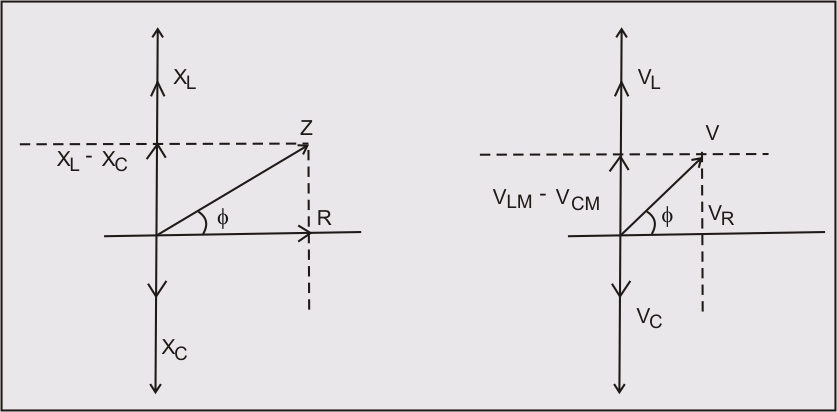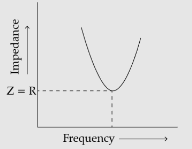# (a)     In a series LCR circuit connected across an ac source of variable frequency, obtain the expression for its impedance and draw a plot showing its variation with frequency of the ac source.(b) What is the phase difference between the voltages across the inductor and the capacitor at resonance in the LCR circuit?(c) When an inductor is connected to a dc voltage, a current of flows through it. When the same inductor is connected to a  ,   ac source, only current flows. Explain, why? Also, calculate the self-inductance of the inductor.

(a)     Let us consider,

a resistance of resistance , an inductor of inductance L, and a capacitor of capacitance C be connected in series across an ac source of frequency f.

We know that the potential difference across the resistor is given by :-

Now, Potential Difference across the capacitor is given by :-

Where,  is the maximum potential across the capacitor

Drawing the phasor diagramThus, By adding it, we can obtain the potential difference across the three components.

On substituting,

and

So, we get

Impedance is explained as

Hence,(b) The phase difference between the voltage across inductor and capacitor is . This can be viewed in the phasor diagram.

(c) In the DC circuit, the resistance is given by:-

When the inductor is connected to ac circuit an additional resistance is offered due to self-inductance of the circuit.

The impedance

### Preparation Products

##### JEE Main Rank Booster 2021

This course will help student to be better prepared and study in the right direction for JEE Main..

₹ 13999/- ₹ 9999/-
##### Rank Booster NEET 2021

This course will help student to be better prepared and study in the right direction for NEET..

₹ 13999/- ₹ 9999/-
##### Knockout JEE Main April 2021 (Subscription)

An exhaustive E-learning program for the complete preparation of JEE Main..

₹ 4999/-
##### Knockout NEET May 2021

An exhaustive E-learning program for the complete preparation of NEET..

₹ 22999/- ₹ 14999/-